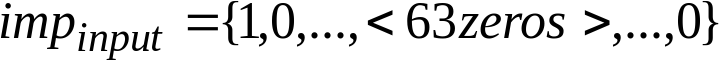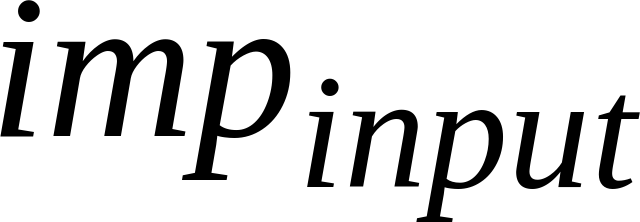## 5.2.5 LPC gain compensation

26.4473GPPCodec for Enhanced Voice Services (EVS)Error concealment of lost packetsRelease 17TS

At 9.6, 16.4, 24.4, 48, 96 and 128 kbps, the LPC concealment and interpolation will lead to a change of overall gain of the signal, which is unwanted when targeting a certain background noise level during consecutive frame loss. Therefore the energy of the LPC is measured and stored during decoding of regular frames. In a concealment frame the energy of the concealed LPC is measured and compared to the LPC energy of the last correctly received frame and any change is compensated.

To measure the LPC energy, a vector of length 64 is generated and initialized to all zero. Then the first entry is set to one:(25)is fed into the LPC synthesis filter, where the filter memory is initialized with zeros. The output of the filter (impulse response) is denoted as. After filtering, the root mean square energy is calculated by:

(26)

In correctly received frames the energy is calculated and stored using the latest LPC available.

In case of concealment the compensation differs for ACELP and TCX:

For ACELP there will be 4 or 5 sets of LPC coefficients, depending on the number of subframes to be synthesized. For each set of coefficients the corresponding energy is calculated and divided by the energy derived in the last good frame. The result of the division is used as a factor to be multiplied to each element of the excitation vector of the corresponding subframe. See sub-clause 5.3.4.2.1.

For TCX, there will be one or two sets of coefficients (TCX10/TCX20). For each set of coefficients the corresponding energy is calculated and divided by the energy derived in the previous segment. The segment size equals 10 ms for TCX10 and 20 ms for TCX20. As the fade out is performed in the time domain, the LPC gain compensation is also done in the time domain, by linearly fading from the last compensation factor (would be 1 for the first lost frame) to the derived compensation factor at the end of the segment. See sub-clause 5.4.6.1.3.